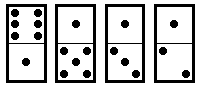# CEOI 97 - Nowy Sącz

Problem: Dominoes

A domino is a flat, thumbsized tile, the face of which is divided into two squares, each left blank or bearing from one to six dots. There is a row of dominoes laid out on a table:The number of dots in the top line is 6+1+1+1=9 and the number of dots in the bottom line is 1+5+3+2=11. The gap between the top line and the bottom line is 2. The gap is the absolute value of difference between two sums.

Each domino can be turned by 180 degrees keeping its face always upwards.

What is the smallest number of turns needed to minimise the gap between the top line and the bottom line?

For the figure above it is sufficient to turn the last domino in the row in order to decrease the gap to 0. In this case the answer is 1.

Write a program that:

• reads the number of dominoes and their descriptions from the text file DOM.IN,
• computes the smallest number of turns needed to minimise the gap between the top line and the bottom line,
• writes the result to the text file DOM.OUT.

Input

The first line of the text file DOM.IN contains an integer n, 1 <= n <= 1000. This is the number of dominoes laid out on the table.

Each of the next n lines contains two integers a, b separated by a single space, 0 <= a, b <= 6. The integers a and b written in the line i + 1 of the input file, 1 <= i <= 1000, are the numbers of dots on the i-th domino in the row, respectively, in the top line and in the bottom one.

Output

Your program should write exactly one integer to the first line of the text file DOM.OUT; this integer should be the smallest number of turns needed to minimise the gap between the top line and the bottom line.

Example

For the text file DOM.IN:

```4
6 1
1 5
1 3
1 2
```
the correct solution is the text file DOM.OUT:
```1
```